Practical analysis for investment professionals
14 June 2018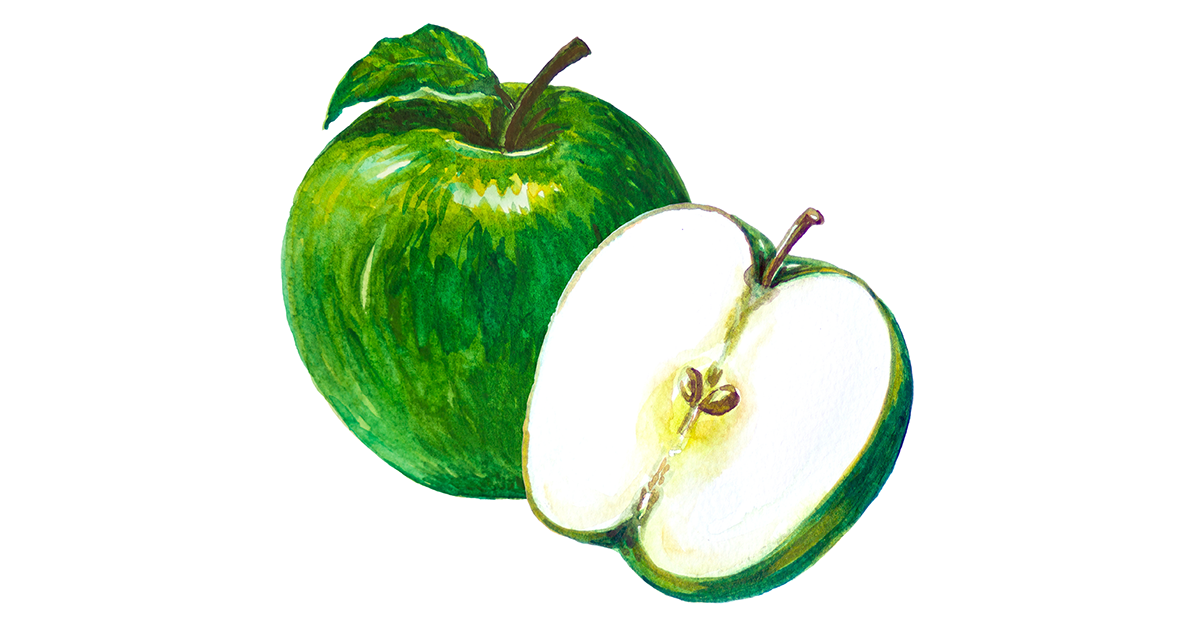# The Kelly Criterion: You Don’t Know the Half of It

Despite expending substantial resources on a formal financial education, I did not encounter the Kelly criterion in business school or the CFA curriculum. I came across it almost by accident, in William Poundstone’s delightful book Fortune’s Formula.

Created in 1956 by John Kelly, a Bell Labs scientist, the Kelly criterion is a formula for sizing bets or investments from which the investor expects a positive return. It is the only formula I’ve seen that comes with a mathematical proof explaining why it can deliver higher long-term returns than any alternative.

In my view, the formula is consistent with the value investing concept of a margin of safety and leads to concentrated portfolios in which the dominant ideas have the greatest edge and smallest downside.Despite its relative obscurity and lack of mainstream academic support, the Kelly criterion has attracted some of the best-known investors on the planet, Warren Buffett, Charlie Munger, Mohnish Pabrai, and Bill Gross, among them. While the Kelly formula requires an estimate of the probability distribution of investment outcomes ahead of time, i.e., a crystal ball, its mainstream alternative, Harry Markowitz’s mean/variance optimization, calls for an estimate of the covariance matrix, which for a bottom-up investor, I believe is much more difficult to obtain.

After reading Poundstone’s book, I wanted to apply the Kelly criterion to my own investing. I learn by example and my math is rusty, so I looked for a short, non-technical article about how the formula can work in an equity-like investment.

Unfortunately, most of the sources I found use the wrong formula.

The top article in a Google search for “Kelly calculator equity” presents a simple, stylized investment with a 60% chance of gaining and a 40% chance of losing 20% in each simulation. No other outcomes are possible, and the investment can be repeated across many simulations, or periods.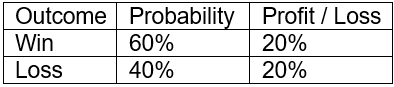It’s clearly a good investment, with a positive expectation: E(x) = 60% * 20 + 40% * (-20%) = 4%. But what share of the portfolio should it take up? Too small an allocation and the portfolio will lose out on growth. Too large and a few unlucky outcomes — even a single one — could depress it beyond recovery or wipe it out altogether. So what percentage allocation, consistently applied, maximizes the portfolio’s potential long-term growth rate?

The article I found and many like it use the formula Kelly % = W – [(1 – W) / R], where W is the win probability and R is the ratio between profit and loss in the scenario.

For this investment, W is 60% and R is 1 (20%/20%). The loss is expressed as a positive. Plugging in the numbers, the Kelly % = 60% – [(1 – 60%) / (20%/20%)] = 20%. In other words, a 20% allocation to the investment maximizes the portfolio’s potential long-term growth.

This is simply incorrect. The error is intuitive, empirical, and mathematical. The formula does not account for the magnitude of potential profits and losses (volatility), only their ratio to each other. Indeed, the article does not even list the potential gain or loss. Change the potential profit and loss from 20% each to 200% each, and the investment becomes 10 times more volatile. Yet the ratio R stays the same — 200%/200% = 1 — as does the formula’s resulting 20% optimal allocation.Consider a simulation with three different allocation scenarios, all replicating the same investment over and over: Red allocates 20% of the portfolio, as the articles suggests, Blue goes all in at 100%, and Green levers up to 150%. The chart below visualizes how the simulation plays out after 100 rounds.In the Red, “Kelly optimal” scenario, a 20% allocation earned a relatively puny 2x return. The Blue, all-in option generated a 6.2x return. Green outpaced Blue for a time but a string of losses in the later rounds led to a 3.4x return.

This wasn’t just a lucky outcome for Blue. Run the simulation 1,000 times and Blue beats Red 79% and Green 67% of the time. Blue’s median return was at least 3x better than Red’s and almost 2x better than Green’s. In short, the 20% allocation is too conservative and the Green option too aggressive.

Ending Portfolio Value after 1,000 Simulations (In Dollars, Starting with \$1 in Period 1)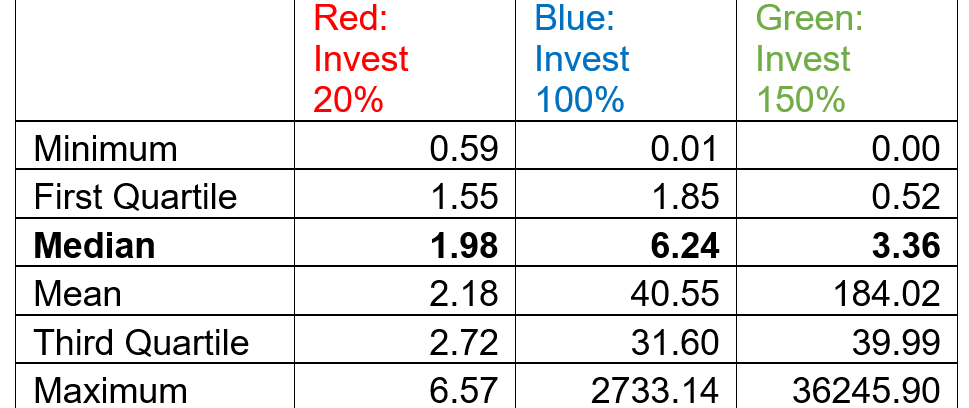The Kelly formula in the first scenario — Kelly % = W – [(1 – W)/R] — is not an anomaly. It turns up in many other sources, including NASDAQMorningstar, Wiley’s For Dummies series, Old School Value, etc., and is analogous to the one in Fortune’s FormulaKelly % = edge/odds.

But the formula works only for binary bets where the downside scenario is a total loss of capital, as in -100%. Such an outcome may apply to blackjack and horse racing, but rarely to capital markets investments.

If the downside-case loss is less than 100%, as in the scenario above, a different Kelly formula is required: Kelly % = W/A – (1 – W)/B, where W is the win probability, B is the profit in the event of a win (20%), and A is the potential loss (also 20%).

Plugging in the values for our scenario: Kelly % = 60%/20% – (1 – 60%)/20% = 100%, which was Blue’s winning allocation.

The theoretical downside for all capital market investments is -100%. Bad things happen. Companies go bankrupt. Bonds default and are sometimes wiped out. Fair enough.

But for an analysis of the securities in the binary framework implied by the edge/odds formula, the downside-scenario probability must be set to the probability of a total capital loss, not the much larger probability of some loss.

There are many criticisms of the Kelly criterion. And while most are beyond the scope of this article, one is worth addressing. A switch to the “correct” Kelly formula — Kelly % = W/A – (1 – W)/B — often leads to significantly higher allocations than the more popular version.

Most investors won’t tolerate the volatility and resulting drawdowns and will opt to reduce the allocation. That’s well and good — both variations of the formula can be scaled down — but the “correct” version is still superior. Why? Because it explicitly accounts for and encourages investors to think through the downside scenario.

And in my experience, a little extra time spent thinking about that is richly rewarded.#### Appendix: Supporting Math

Here is a derivation of the Kelly formula: An investor begins with \$1 and invests a fraction (k) of the portfolio in an investment with two potential outcomes. If the investment succeeds, it returns B and the portfolio will be worth 1 + kB. If it fails, it loses A and the portfolio will be worth 1 – kA.

The investment’s probability of success is w. The investor can repeat the investment as often as desired but must invest the same fraction (k) each time. What fraction k will maximize the portfolio in the long term?

In the long term, after n times where n is large, the investor is expected to have w * n wins and (1 – w)n losses. The portfolio P will be worth: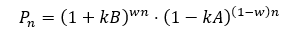We would like to solve for the optimal k: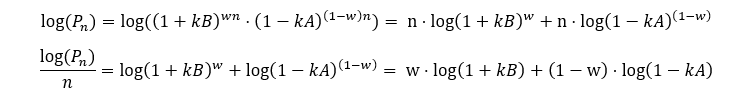To maximize , we take its derivative with respect to k and set it to 0:Solving for k:Note that if the downside-scenario loss is total (A = 1), this formula simplifies to the more popular version quoted above because R = B/A = B/1 = B, so:#### Appendix: Supporting Code

Below is the R code used to produce the simulation and the charts above.

##########################################################
#Kelly Simulation, Binary Security
# by Alon Bochman
##########################################################
trials = 1000 # Repeat the simulation this many times
periods = 100 # Periods per simulation
winprob = 0.6 # Win probability per period
returns = c(0.2,-0.2) # Profit if win, loss if lose
fractions = c(0.2,1,1.5) # Competing allocations to test

library(ggplot2)
library(reshape2)
library(ggrepel)
percent <- function(x, digits = 2, format = “f”, …) {
paste0(formatC(100 * x, format = format, digits = digits, …), “%”)
}

set.seed(136)
wealth = array(data=0,dim=c(trials,length(fractions),periods))
wealth[,,1] =1 #Eq=1 in period 1

#Simulation loop
for(trial in 1:trials) {
outcome = rbinom(n=periods, size=1, prob=winprob)
ret = ifelse(outcome,returns,returns)
for(i in 2:length(ret)) {
for(j in 1:length(fractions)) {
bet = fractions[j]
wealth[trial,j,i] = wealth[trial,j,i-1] * (1 + bet * ret[i])
}
}
}

#Trial 1 Results
view.trial = 1
d <- melt(wealth)
colnames(d) = c(‘Trial’,’Fraction’,’Period’,’Eq’)
d = subset(d,Trial ==view.trial)
d\$Fraction = as.factor(d\$Fraction)
levels(d\$Fraction) = paste(“Invest “,percent(fractions,digits=0),sep=”)
d[d\$Period == periods,’Label’] = d[d\$Period == periods,’Eq’]
ggplot(d, aes(x=Period,y=Eq, col=Fraction)) +
geom_line(size=1) + scale_y_log10() +
labs(y=”Portfolio Value”,x=”Period”) +
guides(col=guide_legend(title=”Allocation”)) +
theme(legend.position = c(0.1, 0.9)) +
scale_color_manual(values=c(“red”, “blue”,”green”)) + #Adjust if >2 allocations
geom_label_repel(aes(label = round(Label, digits = 2)),
nudge_x = 1, show.legend = F, na.rm = TRUE)

#All-Trial Results
d = data.frame(wealth[,,periods]) #Last period only
colnames(d) = paste(“Invest “,percent(fractions,digits=0),sep=”)
summary(d)
nrow(subset(d,d[,2] > d[,1])) / trials #Blue ahead of red
nrow(subset(d,d[,2] > d[,3])) / trials #Blue ahead of green

If you liked this post, don’t forget to subscribe to the Enterprising Investor.

All posts are the opinion of the author. As such, they should not be construed as investment advice, nor do the opinions expressed necessarily reflect the views of CFA Institute or the author’s employer.

Image credit: ©Getty Images/ PATCHARIN SIMALHEK

Share On
##### Alon Bochman, CFA

Alon Bochman, CFA, is a partner in Genpact's (NYSE: G) capital markets consulting practice, based in New York. He works with asset managers and banks to help them make better decisions with data. Previously, he spent two years managing an equity portfolio for SC Fundamental. Bochman began his career as a programmer by co-founding a social networking software firm eventually acquired by Thomson-Reuters. He holds an MBA from Columbia Business School and a BA from the University at Albany.

## 18 thoughts on “The Kelly Criterion: You Don’t Know the Half of It”

1.gregor says:

The general case, wherein the same result as yours is derived, is discussed in the Wikipedia entry for the Kelly criterion.

https://en.wikipedia.org/wiki/Kelly_criterion

2.Alon Bochman says:

Thanks Gregor. Wikipedia has it right. Most other sites – even some professionals – got the formula wrong.

3.JV Miller says:

In 1997 my father famously wrote the article “Debunking the Kelly Criterion.” Sports bettors and investors alike stand to gain a lot of wisdom from the article. https://professionalgambler.com/debunking.html

1.Dwight says:

Mr. Miller, I have your book but it is sorely in need of updating. The latest edition is over 14 years old. I wish you would release a new edition or version because the info is critically outdated.Thank you for your time.

4.Mark Weidmann says:

I am confused by your article. I am either misunderstanding something, or your article is incorrect. The point of the Kelly Criterion is, if you know the correct value of the inputs, the output will give you the optimum percentage of your Total funds to invest. In the example you gave, the Kelly formula said to bet 20%. However, you said it is more optimal to bet 100%. But if you bet 100%, if you lose once, you are broke, and can’t bet again. So, I don’t see why your charts doesn’t show the bet of 100% flatlining to 0 after 1 loss (same with betting 150%).
If you have a positive expected value for a bet, betting 100% will always yield a better expected return than betting 20%, but the problem, or issue is, after one bet you will be broke, and not be able to ever bet anything again.

If you bet 100%, one loss and you are broke. Same with betting 150%.

1.Alon Bochman says:

If you bet 100% and lose, you are not broke because in this scenario, your loss is -20%, not -100%. See the payoff table near the top of the article. This is typical of several capital markets investments, not so much in Blackjack.

5.Brian Richardson says:

What happens if the loss is only 10%, all other parameters remain the same?
You get an expected value of 8% but doesn’t the Kelly% turn negative or have I miscalculated. If so what does it mean ?

1.Andy Klieber says:

A Kelly% equal to or below zero means you dont have a positive expectation and should thus not bet anything at all! So yes, you have likely miscalculated at some point in that case.

1.Pete says:

I read the question as “what happens if you are able to cut the loss shorter at only 10%?”.

Surely this should improve results. I don’t know where the 8% comes from or what the “instead of” original figure was, but clearly a 60% chance of a 20% gain versus a 40% chance of only losing 10% means you’d instead way more.
Here k = 60/10 – 40/20 = 4 meaning you should gear up your investment 4 times (and watch those stop-losses like a hawk), which is what spread-betters and CFD traders aim to do.

The problem in the real world is twofold – first that the leverage comes at a profit-eroding daily cost which is hard to factor in to this form of the equation as it does not have a time element. Second, your 10% loss-limit is much more likely to be hit than if it was a 20% limit so you can’t assume “all other parameters remain the same”.

In theory though there woud be an optimal amount to gear up, but you’d have to keep adjusting it, buying more when in profit and selling when losing, which is what is often done in the real world by geared funds. Whether it is “ideal” to buy on the way up and sell on the way down is another discussion, but Kelly says you “should” to maintain the optimal gearing.

The simulation shown suggests green came out by far the best on average, so would it therefore not be better to have several geared-up separately managed groups of investments that were as uncorrelated as possible, in case of a bad run for one or more of them, rather than just one class of investments with 100% of your money and no gearing?

6.김홍우 says:

Plugging in the values for our scenario: Kelly % = 60%/20% – (1 – 60%)/20% = 100%, which was Blue’s winning allocation.

The correction is
Kelly% = 60%/20%-(1-60%)/20%=20%

1.Matt says:

No YOUR calculation is wrong; that equation does come out to 100%. You can see this by simplifying: 3-2=1 (aka 100%)

7.Aaron says:

Thank you for putting your article together – it raised some thought-provoking points.

The article brings up a few issues with the Kelly Criterion in the application to markets. I’d love to hear your thoughts on these points.
1) Leverage is not infinite so in an example where you wanted to place 5 independent market wagers at 20% bankroll risk and each had 20% downside risk, you would need to have access to at least 5x leverage.

2) The Kelly Criterion assumes you can infinitely divide your minimum bet. Securities markets generally have some minimum wager. With a large enough portfolio, the effect may be close to having the option of infinitely divisible bets but I think it is an important point to call out. How should the Kelly Criterion adjust for the minimum bet size as a % of bankroll?

*My comments are not meant to be investment advice of any kind. I am only looking to add thoughtful discussion to the article.

1.MachineGhost says:

Good points! The reworked formula saves an additional step of figuring out the position size based on the position risk.

8.bruce says:

Hi – I’m trying to run the code.

But it breaks on the first function

percent <- function(x, digits = 2, format = "f", …) {
paste0(formatC(100 * x, format = format, digits = digits, …), “%”)
}

What do the … in the first line of the function mean? I've never seen that before.

Thanks!

1.bruce packard says:

Actually – I figured it out. In my version of R language the quotation marks ” ” and ‘ ‘ are reversed. I’ve had to go through and reverse your usage.
Not sure why that is … I thought this kind of nonsense only happened in different Python versions 🙂

Got it working now!

9.Martin says:

What a waste of time.
Foremostly, you did not even bring the correct formula to the table.

Errors:
1. You modeled the portfolio with discrete probabilities
2. Did account for individual drift rates nor variance rates.
3. No dynamical reallocation between securities and fixed income.

I could go on but I’d be wasting my time on this.

10.Henk Tijms says:

A very interesting article. Indeed the blue strategy maximizes the growth rate of your bankroll in the long run. A more general Kelly formula, which leads to this strategy, is discussed among other practical properties of Kelly betting in Chapter 16 of my book “Surprises in Probability- Seventeen Short Stories, CRC Press, 2019.

11.Axel says:

Thanks a lot for the article. It certainly helps to understand the logic behind the formula…

I think one can argue a lot about the exact numbers here. However, given all the assumptions that go into the calculation I’d see the result more like a rough indication regarding portfolio allocation…

﻿